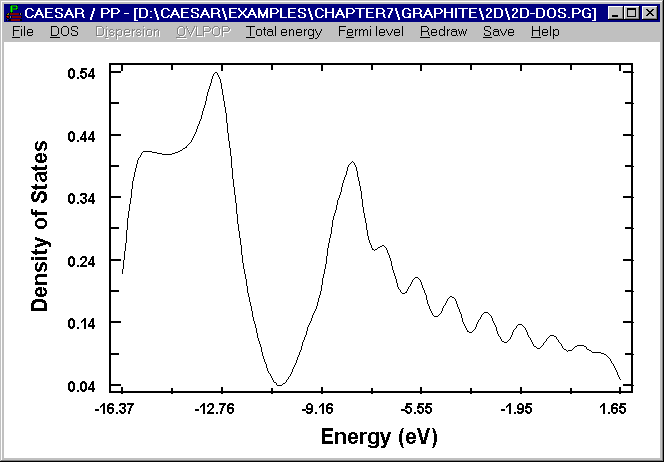# DOS of A Single Graphite Layer

In the CAESAR\Examples\Chapter7\Graphite\2D directory, the structural information of an isolated graphite layer is stored in the 1Gr.CI file. The input files for the BC and PC programs for a single graphite layer are designed to carry out calculations using only the p orbitals. The inputs 2DDsp. BI and 2D-Dsp.PI are used to obtain a dispersion plot, while the 2D-Dos.BI and 2D-Dos.PI are used to obtain a DOS plot. The DOS plot obtained from the above inputs are presented in the following. There are two p electrons in a single graphite layer. Thus the occupied and unoccupied p bands touch at K, and hence the two bands as a whole become a partially filled band. This renders metallic properties to graphite. The DOS plot shows a deep minimum in the region where the Fermi level occurs.Figure. DOS plot calculated for a single graphite layer.

Go back to The Gallery